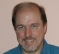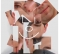## Magnitude of frequency componentsStarted by 6 years ago11 replieslatest reply 6 years ago454 views

Hello all,

I am solving interesting problem at the moment: assume, we have to detect two harmonic components in normal electric network (50 Hz, 230 V RMS). The measure system works wit fs = 2400 Hz, length of buffer is 96 samples, the first component of interest has got frequency of 25 Hz, the second then 75 Hz. We are going to use DFT with k = 1 for 25 Hz and k = 3 for 75 Hz (deltaF = 2400/96 = 25 Hz).

If the grid frequency does not vary (exactly 50 Hz), we will get correct results of component magnitudes and phase shifts. Super!

But if the grid frequency is not stable, the sampling will be not coherent and we will be able to see the influence of frequency leakage in results.

What am I to do with it? What about windowing? OK, if we multiply a length of above mentioned buffer for example 4 times and then use for example Hann window, we will get better results. But unfortunately, the computing load will increase.

Do you know another methods to obtain magnitude of spectral components than with DFT? What about any narrow passband filter or so?

[ - ]Hi electrin,

I don't know a better way than the DFT.  However, I can make some recommendations for your DFT usage and give you formulas for when the frequency varies from your bin values.

This article gives a formula for exact determination of a frequency from DFT bins for a single tone.  In the presence of other tones or noise it is very accurate.  Since the mains shouldn't vary too far from your bin, you should not expect very much interference from the harmonics in this calculation.  Removing the effects of other tones is a future article, but shouldn't be necessary in your case.

Exact Frequency Formula for a Pure Real Tone in a DFT
https://www.dsprelated.com/showarticle/773.php

The optimal calculation occur when you have four samples per cycle.  You have 24 for your 100Hz harmonic, so you are over sampling by a factor of six.  It will greatly reduce your computation load if you use a lower sampling rate.  For instance, using every sixth value, rather than changing your physical setup.

I would spend some of these savings on increasing the sampling interval so your harmonics have larger bin separation in the DFT.  For your current interval the bins of interest are 2 and 4 (Zero indexed, not Matlab indexed).  If you triple your sampling interval the bins of interest will be 6 and 12.  You do not have to calculate the entire DFT, only bins 5,6,7 and 11,12,13.  This will further save calculations.

One you have an estimated frequency for your two harmonics, you can calculate the amplitude and phase value from the DFT bin values using the formulas in this article:

Phase and Amplitude Calculation for a Pure Real Tone in a DFT: Method 1
https://www.dsprelated.com/showarticle/787.php

If the frequency is very close to the bin, then you will want to use just the one bin value as you are currently doing.

If noise is a problem, you can smooth the signal (at your original sampling density), then make the above calculations on the smoothed signal.  The formula for smoothing and recovering the original amplitude from the smoothed amplitude can be found in this article:

Exponential Smoothing with a Wrinkle
https://www.dsprelated.com/showarticle/896.php

For this technique, you will want to calculate the DFT on an interval that is on the interior of the smoothing interval.  The smoothing on the ends is a little distorted.

Hope this helps,
Ced

[ - ]Hi,

I would like to thank you men for your advices. Firstly, it is true that I am really just interested in the magnitude of the energy in bins 1 and 3 in analysed signal - this is exactly my aim. On the other side there is the mentioned problem with varing frequency of second bin (~ 50 Hz). As my first idea to solve it I have chosen DFT and windowing. It works fine, but I need to obtain results a little bit faster.

At the moment I am ruminating about adaptive notch filter to reject ~50 Hz component. But I will test your ideas, too :-)

[ - ]I would not introduce a window here because the current approach is going for exactly one period of 25 Hz in the FFT input buffer.  This period should not be distorted by a window.  Instead I would measure the grid frequency and vary either the sampling rate or the buffer size so that 25 Hz continues to have almost exactly one period in the buffer as intended.

An alternative is to abandon "pitch synchronous" analysis, and take a longer FFT (many cycles), in which case the detection remains easy even when the grid frequency drifts around.

All that said, it should take a lot of deviation from 50 Hz to throw off the harmonic detectors.  The relative amplitudes of k=1,2,3 should remain a pretty stable pattern as the grid rate departs from 50 Hz.  Maybe you can adjust your detection thresholds to tolerate the amount of drift observed in practice.  I assume you are working with the ratios |X(1)/X(2)| and |X(3)/X(2)| for detection.

[ - ]Another idea: reject grid frequency from analysed signal with some filter (notch...) and than use DFT. Hm?

[ - ]I'm sorry, I misunderstood your original question.

I thought you were trying to find the phase and magnitude of the harmonics.

That becomes the first step.  Once you have determined a good estimate of the harmonics you can subtract them from your signal to analyze the rest of the signal.

You can either subtract them in the time domain and take another DFT or your can subtract them from the DFT directly by using the formulas in

DFT Bin Value Formulas for Pure Real Tones
https://www.dsprelated.com/showarticle/771.php

Ced

[ - ]So what is your goal then? To estimate the parameters of your harmonics, to rid your signal of your harmonics, or to suppress the leakage effects of your harmonics? If your goal is the latter and you are concerned with computational efficiency, then windowing to suppress leakage side lobes is your best bet.

[ - ]This just an idea I had, and I'm not an expert so it could be wrong, but here's what I thought:

One way to find out the frequencies of a signal is, if I'm not mistaken, to convolve the audio signal with the frequency you're trying to extract. I also thought I read (but I couldn't find it again) that when you do that, the waves you're convolving with can have any rotation (by rotation i mean kinda how a circular buffer gets rotated). So, I thought, for each frequency you want to extract from the signal, just convolve the signal with a sine wave of the frequency you want, but because it can be rotated and still work, we can take advantage of that by keeping some kind of running sum and just calculating a value for the most recent sample, changing the sum, and then subtracting that part of the sum that's directly in front of the one you just added in the sine wave we're convolving with. If the sine wave can truly be rotated by any amount and still work, then we're effectively rotating it by one sample with each new sample we compute, by simply having a marker where the current front/back of the sine wave is as if it were a circular buffer, without having to do a full convolution for each frequency for each sample.

The Big-O of this would only be [number of samples in the audio] * [number of frequencies you're trying to extract], and you could extract any arbitrary set of frequencies you want and it runs continuously, not block by block.

For the sine waves you can generate a table of sine waves for each frequency you want to extract, or you could just compute them on-demand for each new audio sample. I think the former way would be more efficient.

[ - ]If you're really just interested in the magnitude of the energy in bins 1 and 3 and you know there isn't other interfering energy in those bins to corrupt the measurement, then using something like a flat-top window will make the power measurements in each bin insensitive to small variations in frequency.

Sidelobe leakage can't be avoided entirely if you're using a DFT, but careful selection of a window function may be able to get leakage effects low enough for your application.

A web search on "flat-top window" should get you some decent info.  Depending on how much frequency uncertainty you expect, you may be able to use a less aggressive window and get the same effect.

[ - ]Seems like you're spot on with increasing the buffer length and utilizing a window. Because the bandwidth of each DFT output is going to be more narrow, just perform the calculation less frequently.  Then you shouldn't take as much of a hit on computational load.

If you need a high degree of frequency resolution, I'm not sure you have a choice but to increase the CPU load. If you only need to measure a few harmonic components, then I suppose you could try to dynamically track the fundamental frequency and tune some bandpass frequencies of some filters to the harmonics you want to measure.  That could end up being cheaper from a CPU standpoint.

I wonder if you could dynamically vary the sample rate to track the fundamental such that you always have an integer number of periods in your buffer..

[ - ]You need to reduce the side lobe interference from the other frequency bins when the frequency is not right on, which in the practice never is.

You could implement a WOLA filter to reduce inter-band interference.

With this type of (FFT) every bin will represent the surrounding frequencies with more legitimacy.

As for the computation, other writers are right, you can do computations less often. The WOLA filter bank will require you to do additional buffering and addition, but the FFT size does not change.

You have to pick a fold-this-many-times factor, I worked with 4 or 6 successfully.... and yes, you will have to pick a window too, do not waste time, use Hanning.

[ - ]